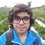# Shortest Distance from Origin - nD

This note is part of the set Shortest Distance from Origin

As work through the problems in this set, you might find a pattern in the formulae of distance of point from line, distance of point from plane and so on. In this note, we will prove that pattern.

Points in one dimensional space, lines in two dimensional space, and planes in there dimensional space are all examples of hyperplanes. A hyperplane in $\mathbb{R}^{n}$ is an $n -1$ dimensional figure, and divides $\mathbb{R}^{n}$ in two halves. It is the set of all points that satisfy

$a_{0} + a_{1} x_{1} + a_{2} x_{2} + a_{3} x_{3} + \cdots + a_{n} x_{n} = 0$

for some real numbers $a_{i}$. For any given point $(b_{1}, b_{2}, b_{3},\ldots, b_{n})$, prove that the shortest distance of a hyperplane in $\mathbb{R}^{n}$ from that point is given by

$d = \frac{|a_{0} + a_{1} b_{1} + a_{2} b_{2} + a_{3}b_{3} + \cdots + a_{n}b_{n}|}{\sqrt{a_{1}^{2} + a_{2}^{2} + a_{3}^{2} + \cdots +a_{n}^{2} }}$Note by Pranshu Gaba
5 years, 1 month ago

This discussion board is a place to discuss our Daily Challenges and the math and science related to those challenges. Explanations are more than just a solution — they should explain the steps and thinking strategies that you used to obtain the solution. Comments should further the discussion of math and science.

When posting on Brilliant:

• Use the emojis to react to an explanation, whether you're congratulating a job well done , or just really confused .
• Ask specific questions about the challenge or the steps in somebody's explanation. Well-posed questions can add a lot to the discussion, but posting "I don't understand!" doesn't help anyone.
• Try to contribute something new to the discussion, whether it is an extension, generalization or other idea related to the challenge.
• Stay on topic — we're all here to learn more about math and science, not to hear about your favorite get-rich-quick scheme or current world events.

MarkdownAppears as
*italics* or _italics_ italics
**bold** or __bold__ bold
- bulleted- list
• bulleted
• list
1. numbered2. list
1. numbered
2. list
Note: you must add a full line of space before and after lists for them to show up correctly
paragraph 1paragraph 2

paragraph 1

paragraph 2

[example link](https://brilliant.org)example link
> This is a quote
This is a quote
    # I indented these lines
# 4 spaces, and now they show
# up as a code block.

print "hello world"
# I indented these lines
# 4 spaces, and now they show
# up as a code block.

print "hello world"
MathAppears as
Remember to wrap math in $$ ... $$ or $ ... $ to ensure proper formatting.
2 \times 3 $2 \times 3$
2^{34} $2^{34}$
a_{i-1} $a_{i-1}$
\frac{2}{3} $\frac{2}{3}$
\sqrt{2} $\sqrt{2}$
\sum_{i=1}^3 $\sum_{i=1}^3$
\sin \theta $\sin \theta$
\boxed{123} $\boxed{123}$

## Comments

Sort by:

Top Newest

Let $\vec{N} = (a_1,a_2,a_3,a_4,\cdots, a_n)$ be a vector in $\mathbb{R}^n$.

Let $\vec{P} = (x_1,x_2,\cdots, x_n)$ be a point in $\mathbb{R}^n$ such that $\vec{P}\cdot\vec{N}$ is always constant. Let this constant be $k (k \in R)$.

Hence, the locus of point $P$ is given by:

$\displaystyle \Rightarrow \vec{P} \cdot \vec{N} = k$

$\displaystyle \Rightarrow \displaystyle \sum_{i=1}^{n}a_i x_i = k$

The above equation represents a hyperplane in $\mathbb{R}^n$ and $\vec{N}$ is the vector normal to the hyperplane.

Comparing it with the equation of given hyperplane yields $k= - a_0$.

Now consider $\vec{B} = (b_1,b_2,\cdots, b_n)$.

The distance of point $B$ from the hyperplane will be measured along the unit vector normal to the hyperplane.

To measure this distance, we find the extent of the join of $B$ and a point $R$ on the hyperplane, along the unit vector normal to the hyperplane. Hence,

$Distance = \left| \vec{BR} \cdot \hat{N} \right|$

$Distance = \left| \frac{ (\vec{R} - \vec{B}) \cdot \vec{N}} {\left| \vec{N} \right| } \right|$

$Distance = \frac{\left| \vec{R} \cdot \vec{N} - \vec{B} \cdot \vec{N} \right|} {\left| \vec{N}\right|}$

$Distance = \frac{\left| k - \displaystyle \sum_{i=1}^n a_i b_i \right|}{\sqrt\sum_{j=1}^n a_j^2}}$

$Distance = \frac{\left| a_1b_1 + a_2b_2 + a_3b_3 + \cdots + a_nb_n - k\right|}{\sqrt{a_1^2 + a_2^2 + \cdots + a_n^2}}$

That is for a general hyperplane. For the given hyperplane, $k=-a_0$.

$\displaystyle \Rightarrow Distance = \frac{\left|a_0 + a_1b_1 + a_2b_2 + \cdots + a_nb_n \right|}{\sqrt{a_1^2 + a_2^2 + \cdots + a_n^2}}$

- 5 years ago

Log in to reply

That's correct. Well done Harsh!

- 5 years ago

Log in to reply

Thank you!

- 5 years ago

Log in to reply

×

Problem Loading...

Note Loading...

Set Loading...